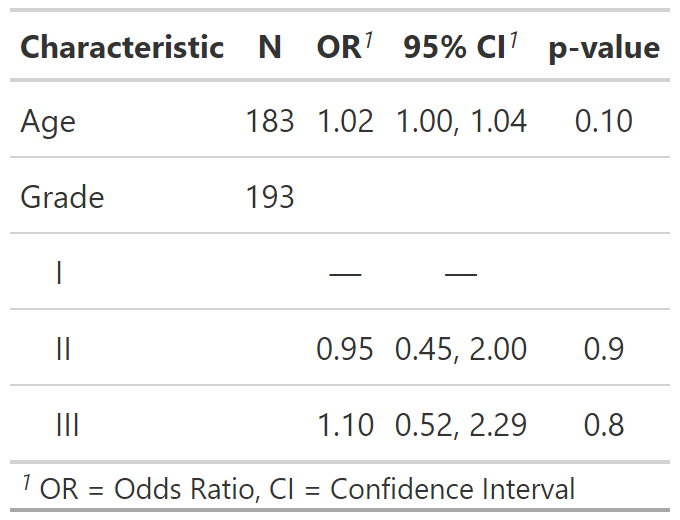This function estimates univariate regression models and returns them in a publication-ready table. It can create univariate regression models holding either a covariate or outcome constant.

For models holding outcome constant, the function takes as arguments a data frame, the type of regression model, and the outcome variable y=. Each column in the data frame is regressed on the specified outcome. The tbl_uvregression function arguments are similar to the tbl_regression arguments. Review the tbl_uvregression vignette for detailed examples.

You may alternatively hold a single covariate constant. For this, pass a data frame, the type of regression model, and a single covariate in the x= argument. Each column of the data frame will serve as the outcome in a univariate regression model. Take care using the x argument that each of the columns in the data frame are appropriate for the same type of model, e.g. they are all continuous variables appropriate for lm, or dichotomous variables appropriate for logistic regression with glm.

tbl_uvregression(
data,
method,
y = NULL,
x = NULL,
method.args = NULL,
exponentiate = FALSE,
label = NULL,
include = everything(),
tidy_fun = NULL,
hide_n = FALSE,
show_single_row = NULL,
conf.level = NULL,
estimate_fun = NULL,
pvalue_fun = NULL,
formula = "{y} ~ {x}",
conf.int = NULL,
...
)

## Arguments

data

Data frame to be used in univariate regression modeling. Data frame includes the outcome variable(s) and the independent variables. Survey design objects are also accepted.

method

Regression method (e.g. lm, glm, survival::coxph, survey::svyglm, and more).

y

Model outcome (e.g. y = recurrence or y = Surv(time, recur)). All other column in data will be regressed on y. Specify one and only one of y or x

x

Model covariate (e.g. x = trt). All other columns in data will serve as the outcome in a regression model with x as a covariate. Output table is best when x is a continuous or dichotomous variable displayed on a single row. Specify one and only one of y or x

method.args

List of additional arguments passed on to the regression function defined by method.

exponentiate

Logical indicating whether to exponentiate the coefficient estimates. Default is FALSE.

label

List of formulas specifying variables labels, e.g. list(age ~ "Age", stage ~ "Path T Stage")

include

Variables to include in output. Input may be a vector of quoted variable names, unquoted variable names, or tidyselect select helper functions. Default is everything().

tidy_fun

Option to specify a particular tidier function for the model. Default is to use broom::tidy, but if an error occurs then tidying of the model is attempted with parameters::model_parameters(), if installed.

hide_n

Hide N column. Default is FALSE

show_single_row

By default categorical variables are printed on multiple rows. If a variable is dichotomous (e.g. Yes/No) and you wish to print the regression coefficient on a single row, include the variable name(s) here--quoted and unquoted variable name accepted.

conf.level

Must be strictly greater than 0 and less than 1. Defaults to 0.95, which corresponds to a 95 percent confidence interval.

estimate_fun

Function to round and format coefficient estimates. Default is style_sigfig when the coefficients are not transformed, and style_ratio when the coefficients have been exponentiated.

pvalue_fun

Function to round and format p-values. Default is style_pvalue. The function must have a numeric vector input (the numeric, exact p-value), and return a string that is the rounded/formatted p-value (e.g. pvalue_fun = function(x) style_pvalue(x, digits = 2) or equivalently, purrr::partial(style_pvalue, digits = 2)).

formula

String of the model formula. Uses glue::glue syntax. Default is "{y} ~ {x}", where {y} is the dependent variable, and {x} represents a single covariate. For a random intercept model, the formula may be formula = "{y} ~ {x} + (1 | gear)".

add a reference value. Default is FALSE

conf.int

Logical indicating whether or not to include a confidence interval in the output. Defaults to TRUE.

...

Not used

## Value

A tbl_uvregression object

## Example Output

Example 1Example 2## Methods

The default method for tbl_regression() model summary uses broom::tidy(x) to perform the initial tidying of the model object. There are, however, a few models that use modifications.

• "parsnip/workflows": If the model was prepared using parsnip/workflows, the original model fit is extracted and the original x= argument is replaced with the model fit. This will typically go unnoticed; however,if you've provided a custom tidier in tidy_fun= the tidier will be applied to the model fit object and not the parsnip/workflows object.

• "survreg": The scale parameter is removed, broom::tidy(x) %>% dplyr::filter(term != "Log(scale)")

• "multinom": This multinomial outcome is complex, with one line per covariate per outcome (less the reference group)

• "gam": Uses the internal tidier tidy_gam() to print both parametric and smooth terms.

• "tidycrr": Uses the tidier tidycmprsk::tidy() to print the model terms.

• "lmerMod", "glmerMod", "glmmTMB", "glmmadmb", "stanreg", "brmsfit": These mixed effects models use broom.mixed::tidy(x, effects = "fixed"). Specify tidy_fun = broom.mixed::tidy to print the random components.

See tbl_regression vignette for detailed examples

Review list, formula, and selector syntax used throughout gtsummary

Other tbl_uvregression tools: add_global_p(), add_q(), bold_italicize_labels_levels, inline_text.tbl_uvregression(), modify, tbl_merge(), tbl_split(), tbl_stack(), tbl_strata()

## Author

Daniel D. Sjoberg

## Examples

# \donttest{
# Example 1 ----------------------------------
tbl_uv_ex1 <-
tbl_uvregression(
method = glm,
y = response,
method.args = list(family = binomial),
exponentiate = TRUE
)

# Example 2 ----------------------------------
# rounding pvalues to 2 decimal places
library(survival)
tbl_uv_ex2 <-
tbl_uvregression(